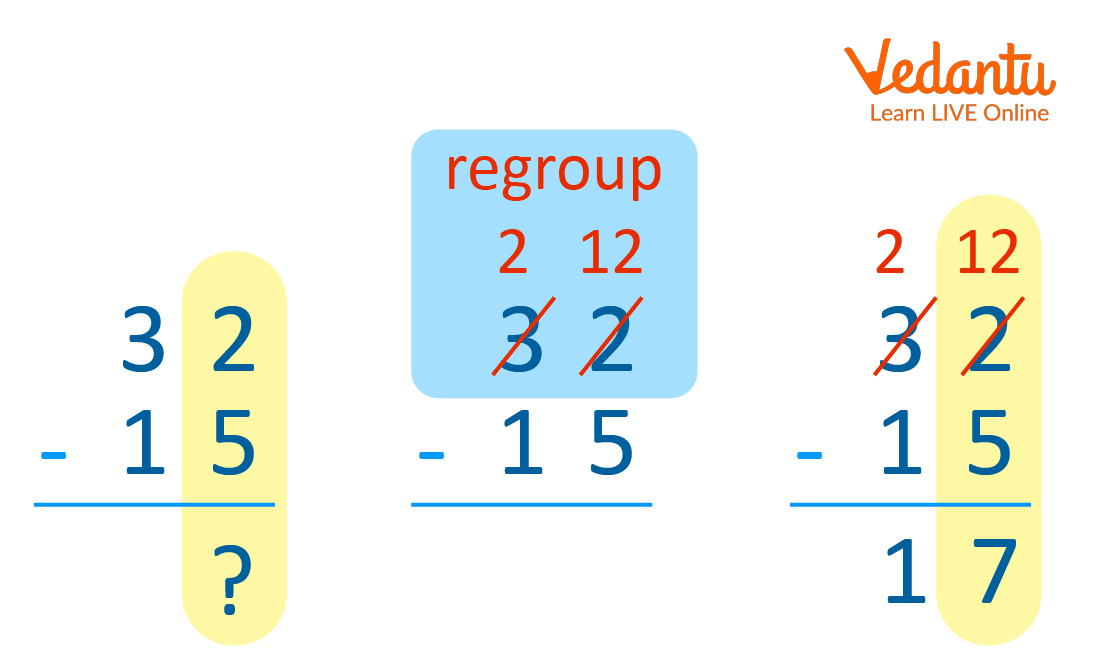Courses
Courses for Kids
Free study material
Free LIVE classes
More

# Regrouping with Addition and SubtractionLIVE
Join Vedantu’s FREE Mastercalss

## What is Regrouping?

Regrouping in Mathematics is defined as the process of making groups of tens when mathematical operations such as addition and subtractions are carried out with two-digit numbers or larger. As the name suggests, to regroup means to rearrange groups in place value to carry out an operation. Regrouping in subtraction is applicable in the situation where numbers in the minuend are smaller than the numbers in the same place in the subtrahend. The regrouping process typically takes place in two-digit numbers.Example of Regrouping

We will understand regrouping with addition step by step with an example.

1. Suppose we want to add together 28 and 22, which equals 50.

2. We should visualise our problem as a grid for better understanding when we are regrouping with addition. Now take a look at the following grid for an example:

 2 8 + 2 2 5 0

3. The first addition here we will perform is to add together 8 and 2, which is equal to 10. But there is no place to write 10 on the bottom row. So, here we will use the regrouping process. In order to perform regrouping, take the 0 from the 10 and place it into the bottom row; after that, take the 1 and place it above the column of ten. Now the updated grid is given below.

 1 2 8 + 2 2 5 0

4. Now the next step is to add the ten’s column. In this case, we would add 1, 2, and 2 together. This brings us to the correct answer to the solution, which is 50.

 1 2 8 + 2 2 5 0

### Regrouping with Subtraction

We will understand regrouping with subtraction step by step with an example similarly to regrouping with addition.

1. Let’s suppose we want to subtract 38 from 66, which equals 28. Again, here we need to put digits into a grid form. The grid is given below.

 6 6 − 3 8 2 8

2. After making the grid set up, we can start regrouping numbers. In the case of subtraction, the process is a little bit different. We need to ‘borrow’ a ten from the first column, so we take a ten from the 60. Now the grid is given below.

 5 16 − 3 8

3. Next, we do the calculations. 16 minus 8 equals 8. For the first column, we do 5−3 = 2. That leaves us with our answer of 28.

 5 16 − 3 8 2 8

### Conclusion

In the above article, we have discussed regrouping. Regrouping is explained with examples and described step by step to perform regrouping with addition and subtraction.

Last updated date: 21st Sep 2023
Total views: 137.7k
Views today: 1.37k

## FAQs on Regrouping with Addition and Subtraction

1. What is meant by regrouping in Maths?

Regrouping in Mathematics can be done by making groups of tens during operations such as subtraction and addition. Regrouping means the rearrangement of numbers into groups by place value to make it easier to carry out operations. This process is called regrouping because we used to rearrange numbers into place values to carry out the process.

2. Why is regrouping important in Maths?

We perform the process of regrouping in doing operations like addition and subtraction to make it easier to work with the digits. Hence, sometimes numbers are easier to work with if we regroup them or arrange them into different groups. Regrouping of numbers is simply to rearrange groups in place value to carry out an operation.